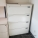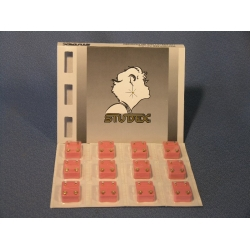CategoriesInformationAllsold.ca BlogShare This Page
|Shopping Cart
0 itemsLoginWanted

Call Dibs on new items!
Looking for Something Else?
Let us know!
You must be logged in to use this feature!Recent PurchasesPanasonic RX-FS430 FM/AM Stereo w Cassette Player \$10.00Blue Boulevard Cubicle System Divider Wall Panels \$140.0036" White Steelcase 4 Drawer Lateral File Cabinet w/ Blonde Top \$325.002 Shelf Rolling Magazine Rack \$25.00Sony CFD-G500 Booombox Stereo with Radio/CD \$65.00

# Studex Ear Piercing StudsClick to enlarge
 Product ID: 1522 Price: \$10.00 Availability: 77

Available Options:
 Style: M101Y (0) Style: M101Y (0) R112Y (0) Style: M101Y (0) R112Y (0) R200W (6) Style: M101Y (0) R112Y (0) R200W (6) R200Y (3) Style: M101Y (0) R112Y (0) R200W (6) R200Y (3) R203Y (0) Style: M101Y (0) R112Y (0) R200W (6) R200Y (3) R203Y (0) R205Y (0) Style: M101Y (0) R112Y (0) R200W (6) R200Y (3) R203Y (0) R205Y (0) R206Y (0) Style: M101Y (0) R112Y (0) R200W (6) R200Y (3) R203Y (0) R205Y (0) R206Y (0) R207Y (0) Style: M101Y (0) R112Y (0) R200W (6) R200Y (3) R203Y (0) R205Y (0) R206Y (0) R207Y (0) R208Y (1) Style: M101Y (0) R112Y (0) R200W (6) R200Y (3) R203Y (0) R205Y (0) R206Y (0) R207Y (0) R208Y (1) R209Y (0) Style: M101Y (0) R112Y (0) R200W (6) R200Y (3) R203Y (0) R205Y (0) R206Y (0) R207Y (0) R208Y (1) R209Y (0) R210Y (0) Style: M101Y (0) R112Y (0) R200W (6) R200Y (3) R203Y (0) R205Y (0) R206Y (0) R207Y (0) R208Y (1) R209Y (0) R210Y (0) R211Y (0) Style: M101Y (0) R112Y (0) R200W (6) R200Y (3) R203Y (0) R205Y (0) R206Y (0) R207Y (0) R208Y (1) R209Y (0) R210Y (0) R211Y (0) R212Y (0) Style: M101Y (0) R112Y (0) R200W (6) R200Y (3) R203Y (0) R205Y (0) R206Y (0) R207Y (0) R208Y (1) R209Y (0) R210Y (0) R211Y (0) R212Y (0) R307Y (1) Style: M101Y (0) R112Y (0) R200W (6) R200Y (3) R203Y (0) R205Y (0) R206Y (0) R207Y (0) R208Y (1) R209Y (0) R210Y (0) R211Y (0) R212Y (0) R307Y (1) R502Y (1) Style: M101Y (0) R112Y (0) R200W (6) R200Y (3) R203Y (0) R205Y (0) R206Y (0) R207Y (0) R208Y (1) R209Y (0) R210Y (0) R211Y (0) R212Y (0) R307Y (1) R502Y (1) R508-4Y (1) Style: M101Y (0) R112Y (0) R200W (6) R200Y (3) R203Y (0) R205Y (0) R206Y (0) R207Y (0) R208Y (1) R209Y (0) R210Y (0) R211Y (0) R212Y (0) R307Y (1) R502Y (1) R508-4Y (1) R111Y (0) Style: M101Y (0) R112Y (0) R200W (6) R200Y (3) R203Y (0) R205Y (0) R206Y (0) R207Y (0) R208Y (1) R209Y (0) R210Y (0) R211Y (0) R212Y (0) R307Y (1) R502Y (1) R508-4Y (1) R111Y (0) R110Y (0) Style: M101Y (0) R112Y (0) R200W (6) R200Y (3) R203Y (0) R205Y (0) R206Y (0) R207Y (0) R208Y (1) R209Y (0) R210Y (0) R211Y (0) R212Y (0) R307Y (1) R502Y (1) R508-4Y (1) R111Y (0) R110Y (0) M110Y (0) Style: M101Y (0) R112Y (0) R200W (6) R200Y (3) R203Y (0) R205Y (0) R206Y (0) R207Y (0) R208Y (1) R209Y (0) R210Y (0) R211Y (0) R212Y (0) R307Y (1) R502Y (1) R508-4Y (1) R111Y (0) R110Y (0) M110Y (0) M111Y (0) Style: M101Y (0) R112Y (0) R200W (6) R200Y (3) R203Y (0) R205Y (0) R206Y (0) R207Y (0) R208Y (1) R209Y (0) R210Y (0) R211Y (0) R212Y (0) R307Y (1) R502Y (1) R508-4Y (1) R111Y (0) R110Y (0) M110Y (0) M111Y (0) M120Y (1) Style: M101Y (0) R112Y (0) R200W (6) R200Y (3) R203Y (0) R205Y (0) R206Y (0) R207Y (0) R208Y (1) R209Y (0) R210Y (0) R211Y (0) R212Y (0) R307Y (1) R502Y (1) R508-4Y (1) R111Y (0) R110Y (0) M110Y (0) M111Y (0) M120Y (1) M200W (0) Style: M101Y (0) R112Y (0) R200W (6) R200Y (3) R203Y (0) R205Y (0) R206Y (0) R207Y (0) R208Y (1) R209Y (0) R210Y (0) R211Y (0) R212Y (0) R307Y (1) R502Y (1) R508-4Y (1) R111Y (0) R110Y (0) M110Y (0) M111Y (0) M120Y (1) M200W (0) M200Y (0) Style: M101Y (0) R112Y (0) R200W (6) R200Y (3) R203Y (0) R205Y (0) R206Y (0) R207Y (0) R208Y (1) R209Y (0) R210Y (0) R211Y (0) R212Y (0) R307Y (1) R502Y (1) R508-4Y (1) R111Y (0) R110Y (0) M110Y (0) M111Y (0) M120Y (1) M200W (0) M200Y (0) M307Y (1) Style: M101Y (0) R112Y (0) R200W (6) R200Y (3) R203Y (0) R205Y (0) R206Y (0) R207Y (0) R208Y (1) R209Y (0) R210Y (0) R211Y (0) R212Y (0) R307Y (1) R502Y (1) R508-4Y (1) R111Y (0) R110Y (0) M110Y (0) M111Y (0) M120Y (1) M200W (0) M200Y (0) M307Y (1) M502Y (1) Style: M101Y (0) R112Y (0) R200W (6) R200Y (3) R203Y (0) R205Y (0) R206Y (0) R207Y (0) R208Y (1) R209Y (0) R210Y (0) R211Y (0) R212Y (0) R307Y (1) R502Y (1) R508-4Y (1) R111Y (0) R110Y (0) M110Y (0) M111Y (0) M120Y (1) M200W (0) M200Y (0) M307Y (1) M502Y (1) R100C/Z (0) Style: M101Y (0) R112Y (0) R200W (6) R200Y (3) R203Y (0) R205Y (0) R206Y (0) R207Y (0) R208Y (1) R209Y (0) R210Y (0) R211Y (0) R212Y (0) R307Y (1) R502Y (1) R508-4Y (1) R111Y (0) R110Y (0) M110Y (0) M111Y (0) M120Y (1) M200W (0) M200Y (0) M307Y (1) M502Y (1) R100C/Z (0) R101Y (0) Style: M101Y (0) R112Y (0) R200W (6) R200Y (3) R203Y (0) R205Y (0) R206Y (0) R207Y (0) R208Y (1) R209Y (0) R210Y (0) R211Y (0) R212Y (0) R307Y (1) R502Y (1) R508-4Y (1) R111Y (0) R110Y (0) M110Y (0) M111Y (0) M120Y (1) M200W (0) M200Y (0) M307Y (1) M502Y (1) R100C/Z (0) R101Y (0) R102Y (0) Style: M101Y (0) R112Y (0) R200W (6) R200Y (3) R203Y (0) R205Y (0) R206Y (0) R207Y (0) R208Y (1) R209Y (0) R210Y (0) R211Y (0) R212Y (0) R307Y (1) R502Y (1) R508-4Y (1) R111Y (0) R110Y (0) M110Y (0) M111Y (0) M120Y (1) M200W (0) M200Y (0) M307Y (1) M502Y (1) R100C/Z (0) R101Y (0) R102Y (0) R103Y (0) Style: M101Y (0) R112Y (0) R200W (6) R200Y (3) R203Y (0) R205Y (0) R206Y (0) R207Y (0) R208Y (1) R209Y (0) R210Y (0) R211Y (0) R212Y (0) R307Y (1) R502Y (1) R508-4Y (1) R111Y (0) R110Y (0) M110Y (0) M111Y (0) M120Y (1) M200W (0) M200Y (0) M307Y (1) M502Y (1) R100C/Z (0) R101Y (0) R102Y (0) R103Y (0) R104Y (0) Style: M101Y (0) R112Y (0) R200W (6) R200Y (3) R203Y (0) R205Y (0) R206Y (0) R207Y (0) R208Y (1) R209Y (0) R210Y (0) R211Y (0) R212Y (0) R307Y (1) R502Y (1) R508-4Y (1) R111Y (0) R110Y (0) M110Y (0) M111Y (0) M120Y (1) M200W (0) M200Y (0) M307Y (1) M502Y (1) R100C/Z (0) R101Y (0) R102Y (0) R103Y (0) R104Y (0) R106Y (0) Style: M101Y (0) R112Y (0) R200W (6) R200Y (3) R203Y (0) R205Y (0) R206Y (0) R207Y (0) R208Y (1) R209Y (0) R210Y (0) R211Y (0) R212Y (0) R307Y (1) R502Y (1) R508-4Y (1) R111Y (0) R110Y (0) M110Y (0) M111Y (0) M120Y (1) M200W (0) M200Y (0) M307Y (1) M502Y (1) R100C/Z (0) R101Y (0) R102Y (0) R103Y (0) R104Y (0) R106Y (0) R107Y (0) Style: M101Y (0) R112Y (0) R200W (6) R200Y (3) R203Y (0) R205Y (0) R206Y (0) R207Y (0) R208Y (1) R209Y (0) R210Y (0) R211Y (0) R212Y (0) R307Y (1) R502Y (1) R508-4Y (1) R111Y (0) R110Y (0) M110Y (0) M111Y (0) M120Y (1) M200W (0) M200Y (0) M307Y (1) M502Y (1) R100C/Z (0) R101Y (0) R102Y (0) R103Y (0) R104Y (0) R106Y (0) R107Y (0) R108Y (0) Style: M101Y (0) R112Y (0) R200W (6) R200Y (3) R203Y (0) R205Y (0) R206Y (0) R207Y (0) R208Y (1) R209Y (0) R210Y (0) R211Y (0) R212Y (0) R307Y (1) R502Y (1) R508-4Y (1) R111Y (0) R110Y (0) M110Y (0) M111Y (0) M120Y (1) M200W (0) M200Y (0) M307Y (1) M502Y (1) R100C/Z (0) R101Y (0) R102Y (0) R103Y (0) R104Y (0) R106Y (0) R107Y (0) R108Y (0) R508Y (1)

Wholesale Cards of 12

Stainless silver and gold Studs and Assorted colored Stones.

Dozens to choose from.

For use with a piercing gun.

• R200W (6)
• R200Y (3)
• R208Y (1)
• R307Y (1)
• R502Y (1)
• R508-4Y (1)
• M120Y (1)
• M307Y (1)
• M502Y (1)
• R508Y (1)

Condition: New

 * Name: * Telephone Number: * E-Mail Address: Enquiry: Enter the code in the box below: